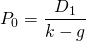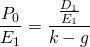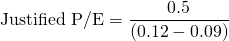# Justified PE

The justified PE ratio or justified price to earnings ratio is a so-called multiple based on fundamentals. This means that, unlike the regular P/E ratio, it is not based on the observed price. Instead, we use inputs for the expected dividend, growth rate, earnings, and cost of equity to estimate the P/E we expect based on future cash flows.

On this page, we explore the justified PE ratio formula, provide a justified PE example, and discuss the limitations of the approach.

## Justified PE formula

To derive the justified PE ratio, we start from the Gordon Growth model:where P0 is the expected stock price, D1 is the expected dividend, k is the required rate of return and g is the expected growth rate of dividends. Now, we divide both sides by expected next year’s earnings E1This PE ratio is a leading P/E ratio since it is based on expected earnings in the future. This is different from the traditional PE ratio, which is based on actual (past) earnings. As a consequence, the traditional PE ratio is a trailing or lagging PE ratio.

The biggest advantage of the justified P/E is that it makes clear how the P/E is related to the company’s fundamentals. In particular, it tells us that:

• D/E = expected dividend payout ratio
• k = required rate of return
• g = expected growth rate of dividends

## Example justified PE ratio

Let’s consider an example to make things clear. Let’s consider a company that has a dividend payout ratio of 50%, a required rate of return of 12%, and a dividend growth rate of 9%. In that case, the justified PE equalsor 16.67.

We can then compare this ratio with the actual PE ratio at which the stock is trading. If the actual PE is below the justified PE, then the company is undervalued. On the other hand, if the actual PE is above 16.67, then the company is overvalued.